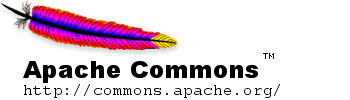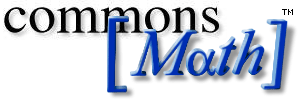## Proposal for math Package

### (0) Rationale

The Java programming language and the math extensions in commons-lang provide implementations for only the most basic mathematical algorithms. Routine development tasks such as computing basic statistics or solving a system of linear equations require components not available in java or commons-lang.

Most basic mathematical or statistical algorithms are available in open source implementations, but to assemble a simple set of capabilities one has to use multiple libraries, many of which have more restrictive licensing terms than the ASF. In addition, many of the best open source implementations (e.g. the R statistical package) are either not available in Java or require large support libraries and/or external dependencies to work.

A commons-math community will provide a productive environment for aggregation, testing and support of efficient Java implementations of commonly used mathematical and statistical algorithms.

### (1) Scope of the Package

The Math project shall create and maintain a library of lightweight, self-contained mathematics and statistics components addressing the most common practical problems not immediately available in the Java programming language or commons-lang. The guiding principles for commons-math will be:

1. Real-world application use cases determine priority
2. Emphasis on small, easily integrated components rather than large libraries with complex dependencies
3. All algorithms are fully documented and follow generally accepted best practices
4. In situations where multiple standard algorithms exist, use the Strategy pattern to support multiple implementations
5. Limited dependencies. No external dependencies beyond Commons components and the JDK

### (1.5) Interaction With Other Packages

math relies only on standard JDK 1.2 (or later) APIs for production deployment. It utilizes the JUnit unit testing framework for developing and executing unit tests, but this is of interest only to developers of the component.

No external configuration files are utilized.

### (2) Initial Source of the Package

The initial codebase will consist of implementations of basic statistical algorithms such as the following:

• Simple univariate statistics (mean, standard deviation, n, confidence intervals)
• Frequency distributions
• t-test, chi-square test
• Random numbers from Gaussian, Exponential, Poisson distributions
• Random sampling/resampling
• Bivariate regression, corellation
and mathematical algorithms such as the following:
• Basic Complex Number representation with algebraic operations
• Newton's method for finding roots
• Binomial coefficients
• Exponential growth and decay (set up for financial applications)
• Polynomial Interpolation (curve fitting)
• Basic Matrix representation with algebraic operations

The proposed package name for the new component is org.apache.commons.math.

### (3) Required Jakarta-Commons Resources

• CVS Repository - New directory math in the jakarta-commons CVS repository.
• Mailing List - Discussions will take place on the general dev@commons.apache.org mailing list. To help list subscribers identify messages of interest, it is suggested that the message subject of messages about this component be prefixed with [math].
• Bugzilla - New component "math" under the "Commons" product category, with appropriate version identifiers as needed.
• Jyve FAQ - New category "commons-math" (when available).

### (4) Initial Committers

The initial committers on the math component shall be: